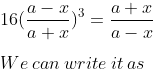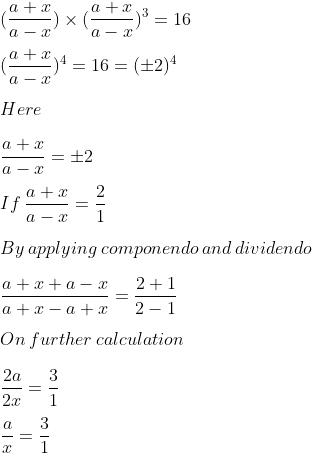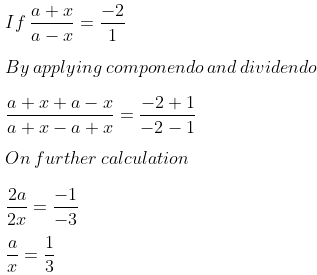Guru

# Solve for x: 16(a−x/a+x)³=a+x/a−x

• 0

This is a basic question from ML aggarwal book of class 10th, chapter 7, ratio and proportion., ICSE board

In this question we have been given a equation with variable x and we have to find the value of x.

Question 13, 7.3

Share

1. Solution:So we get

3x = a

x = a/3So we get

x = 3a

Therefore, x = a/3, 3a.

• 0